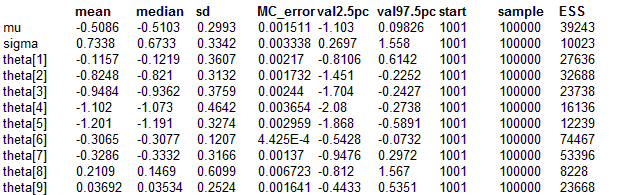#### Preeclampsia: Case control study a two stage approach

We analyse case control data for preeclampsia using a two stage procedure. In the stage one model there is a uniform prior on treatment effect theta[i]. We use the results of this analysis as the input to the second stage analysis.

model {
for (i in 1:N) {
x.C[i] ~ dbin(pi.C[i], n.C[i])
x.T[i] ~ dbin(pi.T[i], n.T[i])
logit(pi.C[i]) <- eta[i] - theta[i]/2
logit(pi.T[i]) <- eta[i] + theta[i]/2
eta[i] ~ dnorm(0, 0.0001)
theta[i] ~ dnorm(0, 0.0001)
}
}
Data

list(
N = 9,
x.C = c(14,17,24,18,35,175,20,2,40),
n.C = c(136,134,48,40,760,1336,524,103,102),
x.T = c(14,21,14,6,12,138,15,6,65),
n.T = c(131,385,57,38,1011,1370,506,108,153))

Inits

list(
eta = c(0,0,0,0,0,0,0,0,0),
theta = c(0,0,0,0,0,0,0,0,0))

Running 1000 iteration burn-in and then 200,000 iterations with thin = 20 gives the following results + samples stored in preeclampsia_stage2_data.odc

For the second stage model we put a normal prior on the theta with unknown mean and precision.

model {
for (i in 1:N) {
for (j in 1 : L) {
samples[i, j] <- data[(i - 1) * L + j]
}
samples[i, 1 : L] ~ dlike.emp(theta[i])
theta[i] ~ dnorm(mu, sigma2.inv)
}
mu ~ dnorm(0, 0.0001)
sigma2.inv <- 1/pow(sigma, 2)
sigma ~ dunif(0, 10)
}

Data
list(L = 10000, N = 9)

plus the CODA file
Data( click to open )
Inits
list(mu = 0, sigma = 1,
theta = c(0,0,0,0,0,0,0,0,0))

Results